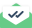# thermodynamic properties

Dear Lammps users,

I used Lammps for investigating the accuracy of an equation of state for different ionic liquids. My simulations predict the PVT data of the substances very accurate compared to experimental data.

actually, the experimental data for thermodynamic properties of ionic liquids that I used are very scarce, therefore, I should compare the total energy and enthalpy with the PVT data which are proved to be correct in compared to experimental data and I used a formula from my equation of state. total energy and enthalpy which are in Lammps log file are very different from E, H that I calculate from Lammps log file PVT. I am sure about the formula of the equation of state and unit conversion.

these are from my input file:

units real
dimension 3
boundary p p p
atom_style full

I used NPT and NVE ensembles data, but E,H are from NPT.Sent with Mailtrack